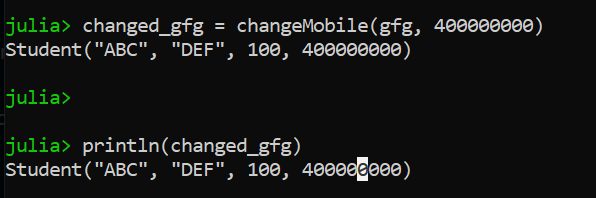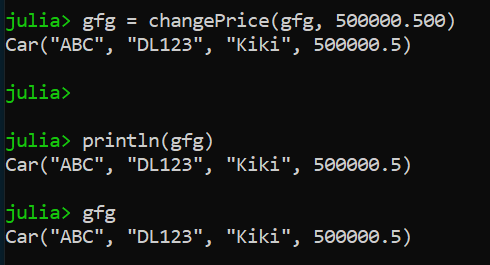# Pure Functions and Modifiers in Julia

• Difficulty Level : Hard
• Last Updated : 25 Aug, 2020

Functional programming is very common nowadays, where we have to write a function to update and modify the variables or do some modifications according to the requirement. In Julia, we have two approaches to update and modify the variables and objects that are Pure Functions and the others are Modifiers let’s see one by one each of them with examples.

#### Pure Functions

When we are working on a function and does not perform any kind of changes by using that object which is passed as an argument. Or we can say that we need to create a separate instance of the same class to update the variable and return the newly updated object.

Let’s say we have a structure of student and that structure has attributes like student name, student roll number, student mobile number, and student address and we are initializing all the variables for demonstration.

## Julia

 `# Create Structure Student ``struct Student``     ``name::String``     ``address::String``     ``roll::Integer``     ``mobile::Integer``end`` ` `# Initialize the Structure Student``gfg ``=` `Student(``"ABC"``, ``"DEF"``, ``100``, ``100000000``)`

Now suppose we have to update the mobile number of the student, we have to make a function name changeMobile where we have to pass the object of student type and other is the new mobile number to get the update and return the new Student type object after updation.

## Julia

 `# Create a Function name changeMobile`` ` `function changeMobile(stud::Student, newmobile::Integer)``      ``name ``=` `stud.name``      ``address ``=` `stud.address``      ``roll ``=` `stud.roll``      ``demo_gfg ``=` `Student(name, address, roll, newmobile)``      ``return` `demo_gfg``end`

As of now, we have created our function. Now we have to use this function to update the mobile number of the existing student while not changing the variables directly.

## Julia

 `# Use the changeMobile function ``changed_gfg ``=` `changeMobile(gfg, ``400000000``)`` ` `println(changed_gfg)`

Output :#### Modifiers

When we are working on a function and does perform any kind of change by using that object which is passed as an argument. Or we can say that we don’t need to create a separate instance of the same class to update the variable and return the same updated object.

Let’s say we have a structure of car and that structure has attributes like car name, car number, car owner’s name, and car price and we are initializing all the variables for demonstration.

## Julia

 `# Create Structure Student ``struct Car``     ``name::String``     ``number::String``     ``owner::String``     ``price::Float64``end`` ` `# Initialize the Structure Car``gfg ``=` `Car(``"ABC"``, ``"DL123"``, ``"Kiki"``, ``45000.00``)`

Now suppose we have to update the price of a car, we have to make a function name changePrice where we have to pass the object of Car type and other is the updated car price to get an update and return the updated same instance of a Car type object.

## Julia

 `# Create a Function name changePrice`` ` `function changePrice(car::Car, newprice::Float64)``      ``name ``=` `car.name``      ``number ``=` `car.number``      ``owner ``=` `car.owner``      ``gfg ``=` `Car(name, number, owner, newprice)``      ``return` `gfg``end`

As of now, we have created our function. Now we have to use this function to update the price of the existing car while not changing the variables directly.

## Julia

 `# Use the changePrice function ``gfg ``=` `changePrice(gfg, ``500000.500``)`` ` `println(gfg)`

Output :Which one methodology we should prefer to write any function?

We always need to prefer the Pure Function methodology because we will not lose our original data and can have updated data too on which we can work. This type of methodology creates functions that are faster to develop and has less possibility of having errors than programs that use modifiers.

My Personal Notes arrow_drop_up